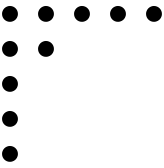#Function Repository Resource:

# ConjugatePartition

Switch the rows and columns of a partition

Contributed by: Wolfram Staff
 ResourceFunction["ConjugatePartition"][p] gives the partition that switches the rows and columns of the integer partition p.

## Details and Options

A partition of n is a list of weakly decreasing positive integers that add up to n. For instance, written compactly, these are the five partitions of 4: 4, 31, 22, 211, 1111.

## Examples

### Basic Examples

Here is a partition of 10 and its Ferrers diagram:

 In:=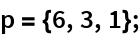In:=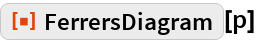Out=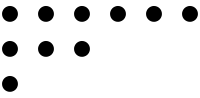Here is the conjugate partition and its Ferrers diagram:

 In:=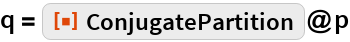Out=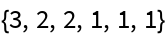In:=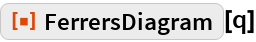Out=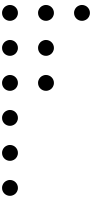Some partitions are self-conjugate:

 In:=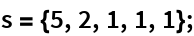In:=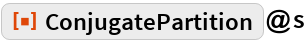Out=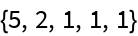A self-conjugate partition has a symmetric Ferrers diagram:

 In:=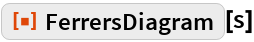Out=